## Regression

### IntroductionMost of the other chapters of our machine learning tutorial with Python are dealing with classification problems. Classification is the task of predicting a discrete class label, whereas regression is the task of predicting a continuous quantity. Some algorithms can be used for both classification and regression, if we apply small modifications: Decision trees and artificial neural networks.

The topics of this chapter will be regression, but what are typcial regression problems?

Typcial regression problems are for example the prediction of

• house prices
• car prices
• exchange rates
• the price of shares

This chapter of our regression tutorial will start with the LinearRegression class of sklearn.

Yet, the bulk of this chapter will deal with the MLPRegressor model from sklearn.neural network. It is a Neural Network model for regression problems. The name is an acronym for multi-layer perceptron regression system. MLP or multi-layer perceptron is an artificial neural network (ANN), which consists of a minimum of three layers:

• an input layer,
• one or more hidden layers and
• an output layer.

Yet, before we start with the MLPRegressor, we will have a look at simpler models.

### Simple Example with Linear Regression

LinearRegression from the sklearnmodule is an ordinary least squares Linear Regression.

LinearRegression fits a linear model with coefficients $w = (w_1, …, w_p)$ to minimize the residual sum of squares between the observed targets in the dataset, and the targets predicted by the linear approximation.

Linear regression is about finding a line of the form

$$y= a \cdot x + b$$

where x is the explanatory variable and y is the dependent variable. The slope of the line is a, and b is the intercept, which corresponds to the value of y if y is equal to 0.

Our first example uses the data of the file regr_example_data1.csv. We will first load the data and separate the data in the X and y values:

import numpy as np

data[:4]

Output:
array([[0. , 2.8],
[0.2, 4.8],
[0.4, 4.4],
[0.6, 5.6]])
X = data[:, 0]
y = data[:, 1]


The data was artificially created. When we created the data, we modified the data created by $4.5 \cdot x + 2.8$. This has been the idealdata and we name it in the following plot as y_opt:

    import matplotlib.pyplot as plt
fig, ax = plt.subplots()

y_opt = 4.5 * X + 2.8
ax.scatter(X, y)
ax.plot(X, y_opt)

Output:
[<matplotlib.lines.Line2D at 0x7fd370d7b7f0>]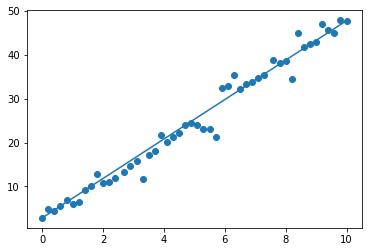We will use now LinearRegression from sklearn to calculate a linear approximation. Of course, we pretend not to know the equation $4.5 \cdot x + 2.8$ with which we had created the test data. We fit the values X to y by using the fit function:

from sklearn.linear_model import LinearRegression
X = X.reshape(X.shape, 1)
reg = LinearRegression().fit(X, y)


We can use the method predict which uses the linear model to predict result. We can apply it to the X values to see, if it works well:

y_predict = reg.predict(X)
y_predict[-10:]

Output:
array([39.6799633 , 40.60471101, 41.52945872, 42.45420642, 43.37895413,
44.30370183, 45.22844954, 46.15319725, 47.07794495, 48.00269266])
help(reg.predict)

Help on method predict in module sklearn.linear_model._base:

predict(X) method of sklearn.linear_model._base.LinearRegression instance
Predict using the linear model.

Parameters
----------
X : array-like or sparse matrix, shape (n_samples, n_features)
Samples.

Returns
-------
C : array, shape (n_samples,)
Returns predicted values.



We get also the value for the intercept and the coefficient:

reg.intercept_, reg.coef_

Output:
(1.7653073394495422, array([4.62373853]))

This means, we can write the straight line equation calculated by the regression:

import matplotlib.pyplot as plt
fig, ax = plt.subplots()

y_opt = X * 4.5 + 2.8
y_reg = X *reg.coef_ + reg.intercept_
ax.scatter(X, y)
ax.scatter(X, y_predict, color="orange")
ax.plot(X, y_opt)
ax.plot(X, y_reg, color="green")

Output:
[<matplotlib.lines.Line2D at 0x7fd36d801fa0>]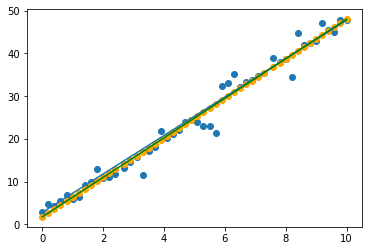### Linear Regression with scipy.stats.linregress

We can also calculate linear regression with the linregress function from the scipy.stats module.

We can use again the data from before and use now linregress:

# we have to reshape X from two dimensional to one-dimensional
X = X.reshape(-1)

from scipy.stats import linregress
slope, intercept, r_value, p_value, std_err = linregress(X, y)


We can visualize the data and the results:

import matplotlib.pyplot as plt
fig, ax = plt.subplots()

ax.scatter(X, y)
ax.plot(X, X*slope + intercept)

Output:
[<matplotlib.lines.Line2D at 0x7fd36d355340>]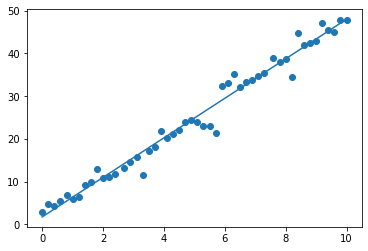## Another Example

We will use some simple artificial data, which gives the price of a car (same model and type) and the age. The file is called car_prices.txt:

from scipy import stats
import numpy as np

years = data[:,0]
prices = data[:,1]
slope, intercept, r_value, p_value, std_err = stats.linregress(years, prices)

import matplotlib.pyplot as plt
fig, ax = plt.subplots()

ax.scatter(years, prices)
ax.plot(years, years*slope + intercept)

Output:
[<matplotlib.lines.Line2D at 0x7fd36d242040>]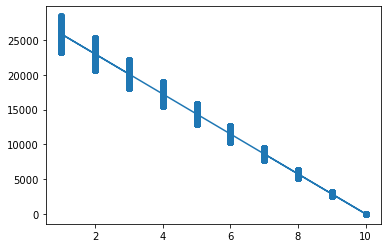import numpy as np
from sklearn.model_selection import train_test_split

from sklearn import datasets, linear_model, metrics

data_sets = train_test_split(years.reshape(-1,1),
prices.reshape(-1,1),
train_size=0.8,
test_size=0.2,
random_state=42)

train_data, test_data, train_targets, test_targets = data_sets
train_data[-5:], train_targets[-5:]

Output:
(array([[10.],
[ 4.],
[ 7.],
[ 7.],
[ 3.]]),
array([[    0.  ],
[18432.36],
[ 7829.35],
[ 9042.8 ],
[18381.84]]))
regr = linear_model.LinearRegression()
regr.fit(train_data, train_targets)
regr.predict(train_data)

Output:
array([[25850.95101352],
[17234.9152252 ],
[ 8618.87943687],
...,
[ 8618.87943687],
[ 8618.87943687],
[20106.92715464]])
train_targets[:20]

Output:
array([[23978.3 ],
[17302.83],
[ 8992.9 ],
[11226.09],
[24430.87],
[ 6158.63],
[    0.  ],
[ 5421.7 ],
[14040.26],
[20246.37],
[    0.  ],
[    0.  ],
[27107.66],
[ 3108.44],
[ 6203.36],
[    0.  ],
[18620.77],
[    0.  ],
[ 5398.64],
[14905.14]])

### Non-linear Example

We use a file car_prices.txt, where each line contains a sample with the age and the value of a car:

8 5332.82
3 12079.80
9 2754.36
4 14382.52
from scipy import stats
import numpy as np

years = data[:, 0]
prices = data[:, 1]

data_sets = train_test_split(years.reshape(-1,1),
prices,
train_size=0.8,
test_size=0.2,
random_state=42)
print(years.shape)
train_data, test_data, train_targets, test_targets = data_sets

(4999,)

import matplotlib.pyplot as plt
fig, ax = plt.subplots()

ax.scatter(years, prices)

Output:
<matplotlib.collections.PathCollection at 0x7fd36d29c700>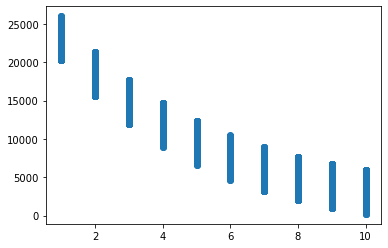from sklearn.neural_network import MLPRegressor

clf = MLPRegressor(solver='lbfgs',
alpha=1e-5,     # used for regularization, ovoiding overfitting by penalizing large magnitudes
hidden_layer_sizes=(5, 2), random_state=24)
clf.fit(train_data, train_targets)
res = clf.predict(train_data)

res

Output:
array([ 8769.29762279,  2866.74419242,  3994.62057336, ...,
7378.24971619, 22411.61185359,  5122.49695431])

An explanation of the parameters of MLPRegressor comes further down in this chapter.

predictions = clf.predict(train_data)
predictions[:10]

Output:
array([ 8769.29762279,  2866.74419242,  3994.62057336, 15590.45473819,
6250.37333525,  3994.62057336, 19001.03329589,  2866.74419242,
8769.29762279,  6250.37333525])
train_targets[:10]

Output:
array([ 9416.01,  4320.32,  4813.04, 15306.8 ,  5293.85,  3922.08,
19262.51,  3427.23,  9502.91,  8615.61])

### Create Regression Test Data

import matplotlib.pyplot as plt
from sklearn.datasets import make_regression
data, targets = make_regression(n_samples=100,
n_features=1,
noise=0.1)

data[:5]

Output:
array([[ 1.18953199],
[ 1.33455338],
[ 1.15627395],
[-0.27968365],
[ 0.24416149]])
from sklearn import datasets

plt.scatter(data, targets)
plt.show()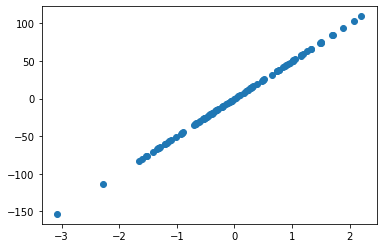import matplotlib.pyplot as plt
from sklearn.datasets import make_regression
data, targets = make_regression(n_samples=100,
n_features=3,
#shuffle=True,
noise=0.1)

data[:5]

Output:
array([[-0.62737501,  1.05747196, -0.16250019],
[ 0.60361949, -0.48402074, -0.21411057],
[ 0.70154627,  0.80708778,  0.37729884],
[ 0.70548775,  0.33157616, -0.00889534],
[ 0.00878579,  1.68153764,  1.27386594]])
import pandas as pd
data_df = pd.DataFrame(data)
data_df.insert(len(data_df.columns),
column="result",
value=targets)
data_df.columns = "blue", "green", "red", "result"


Output:
blue green red result
0 -0.627375 1.057472 -0.162500 39.624626
1 0.603619 -0.484021 -0.214111 -3.926592
2 0.701546 0.807088 0.377299 146.887520
3 0.705488 0.331576 -0.008895 87.969682
4 0.008786 1.681538 1.273866 207.650988
from sklearn.model_selection import train_test_split
data_sets = train_test_split(data,
targets,
test_size=0.30,
random_state=42)

data_train, data_test, targets_train, targets_test = data_sets

clf = MLPRegressor(solver='lbfgs',        #  ‘lbfgs’, ‘sgd’, ‘adam’ (default)
alpha=1e-5,            # used for regularization, ovoiding overfitting by penalizing large magnitudes
hidden_layer_sizes=(3, 1),
activation='logistic', # ‘identity’, ‘logistic’, ‘tanh’, ‘relu’ (default)
max_iter=10000,
random_state=42)
clf.fit(data_train, targets_train)
clf.predict(data_train)

Output:
array([-101.67243732, -101.67243732, -101.67243732,   90.72634183,
-101.67243732, -101.67243732,  -58.45108971, -101.67243732,
-101.67243732,   90.72698154,   90.72633911, -101.67243732,
90.72698155,   90.72634671,   90.72634673,   90.72696989,
90.72634543, -101.67243731, -101.67243732, -101.67242528,
-101.67243731,   90.72653465, -101.67243732, -101.67243732,
-101.67243732,   90.72634673, -101.67243732,   90.72698061,
-101.67243732, -101.67243732, -101.67243732,   90.72634673,
90.72695303, -101.67243732,  -99.56032534, -101.67243732,
-101.67243732,   -0.52219877, -101.67243732, -101.67243732,
90.72634673,   79.39810386, -101.67243732, -101.67243731,
-101.67243732,  -74.3548856 , -101.67243731,   90.72634692,
-101.67243732,   90.72634671,   90.72634673,   90.72634673,
90.72634673, -101.67243732, -101.67243732, -101.67243732,
-101.67243732,   90.72693198, -101.67243732,   90.72698154,
-101.67243732, -101.67243732,   90.72634572,    7.76950713,
90.72634673,   90.72698155, -101.67243732,   90.72698153,
90.72633639,   90.72634733])

### California-Housing-DatasetNow, we will use a dataset derived from the 1990 U.S. census. The data is solely from California. The data is organized as one row per census block group. A block group is the smallest geographical unit for which the U.S. Census Bureau publishes sample data (a block group typically has a population of 600 to 3,000 people).

The dataset contains 20640 samples. Each sample with 8 numeric, predictive attributes and the target value.

The attributes are:

• MedInc: median income in block
• HouseAge: median house age in block
• AveRooms: average number of rooms
• AveBedrms: average number of bedrooms
• Population: block population
• AveOccup: average house occupancy
• Latitude: house block latitude
• Longitude: house block longitude

This dataset was obtained from the StatLib repository: http://lib.stat.cmu.edu/datasets/

learn.datasets.fetch_california_housing

We download the California housing dataset in the following Python code lines:

from sklearn.datasets import fetch_california_housing
dataset = fetch_california_housing()


Let us look at the feauture names:

feature_names = dataset['feature_names']
print("Feature names: {}\n".format(feature_names))

Feature names: ['MedInc', 'HouseAge', 'AveRooms', 'AveBedrms', 'Population', 'AveOccup', 'Latitude', 'Longitude']



As usual the data contains the data for the samples. Each line contains eight float values, which correspond to the features:

print("number of samples in the file (number of rows): ", dataset.data.shape)
print("number of features per row (columns): ", dataset.data.shape)
dataset.data[:4]

number of samples in the file (number of rows):  20640
number of features per row (columns):  8

Output:
array([[ 8.32520000e+00,  4.10000000e+01,  6.98412698e+00,
1.02380952e+00,  3.22000000e+02,  2.55555556e+00,
3.78800000e+01, -1.22230000e+02],
[ 8.30140000e+00,  2.10000000e+01,  6.23813708e+00,
9.71880492e-01,  2.40100000e+03,  2.10984183e+00,
3.78600000e+01, -1.22220000e+02],
[ 7.25740000e+00,  5.20000000e+01,  8.28813559e+00,
1.07344633e+00,  4.96000000e+02,  2.80225989e+00,
3.78500000e+01, -1.22240000e+02],
[ 5.64310000e+00,  5.20000000e+01,  5.81735160e+00,
1.07305936e+00,  5.58000000e+02,  2.54794521e+00,
3.78500000e+01, -1.22250000e+02]])

### A Closer Look at the California Housing Data

As we have already mentioned, each sample (row) of the data corresponds to a block (district). A clock contains an unspecified number of houses. The second column (index == 1) contains the median house age of the block. We can filter the data by looking at the blocks with an avarage age less than 10 years:

n = 10   # median house age in a block
# data where the houses in the block are less than 10 years old:
dataset.data[dataset.data[:,1]<n]

Output:
array([[   2.5625    ,    2.        ,    2.77192982, ...,    1.64912281,
37.82      , -122.29      ],
[   7.611     ,    5.        ,    6.85577631, ...,    2.73252391,
37.72      , -122.24      ],
[   7.0568    ,    5.        ,    7.0234375 , ...,    3.39453125,
37.73      , -122.06      ],
...,
[   3.4186    ,    6.        ,    5.66256158, ...,    3.15394089,
38.53      , -121.99      ],
[   2.0474    ,    5.        ,    4.37813212, ...,    2.13667426,
39.15      , -121.59      ],
[   3.        ,    5.        ,    6.06779661, ...,    2.86440678,
39.13      , -121.32      ]])

The target variable is the median house value for California districts:

dataset.target

Output:
array([4.526, 3.585, 3.521, ..., 0.923, 0.847, 0.894])

### Visualizing the Data

We can get a better view on the data by turning it into a Pandas DataFrame:

import pandas as pd
data_df = pd.DataFrame(dataset.data)

data_df.columns = ["MedInc", "HouseAge", "AveRooms",
"AveBedrms", "Population", "AveOccup",
"Latitude", "Longitude"]


Output:
MedInc HouseAge AveRooms AveBedrms Population AveOccup Latitude Longitude
0 8.3252 41.0 6.984127 1.023810 322.0 2.555556 37.88 -122.23
1 8.3014 21.0 6.238137 0.971880 2401.0 2.109842 37.86 -122.22
2 7.2574 52.0 8.288136 1.073446 496.0 2.802260 37.85 -122.24
3 5.6431 52.0 5.817352 1.073059 558.0 2.547945 37.85 -122.25
4 3.8462 52.0 6.281853 1.081081 565.0 2.181467 37.85 -122.25

We will insert now the average house value to our DataFrame:

data_df.insert(loc=len(data_df.columns),
column="AvePropVal",
value=dataset.target.reshape(-1,1))

data_df[:5]

Output:
MedInc HouseAge AveRooms AveBedrms Population AveOccup Latitude Longitude AvePropVal
0 8.3252 41.0 6.984127 1.023810 322.0 2.555556 37.88 -122.23 4.526
1 8.3014 21.0 6.238137 0.971880 2401.0 2.109842 37.86 -122.22 3.585
2 7.2574 52.0 8.288136 1.073446 496.0 2.802260 37.85 -122.24 3.521
3 5.6431 52.0 5.817352 1.073059 558.0 2.547945 37.85 -122.25 3.413
4 3.8462 52.0 6.281853 1.081081 565.0 2.181467 37.85 -122.25 3.422

There are blocks where the average number of rooms is more than a hundred. Not very likely? At least the average number of bedrooms correlates:

data_df[data_df['AveRooms']>100]

Output:
MedInc HouseAge AveRooms AveBedrms Population AveOccup Latitude Longitude AvePropVal
1914 1.875 33.0 141.909091 25.636364 30.0 2.727273 38.91 -120.10 5.00001
1979 4.625 34.0 132.533333 34.066667 36.0 2.400000 38.80 -120.08 1.62500

We can assume that the samples (districts) where the average number of rooms is larger than 11 must be rubbish. So we have a look at the data where the number is less than 12:

no_of_districts_before_cleansing = len(data_df)
data_df = data_df[data_df['AveRooms']<12]

data_df

Output:
MedInc HouseAge AveRooms AveBedrms Population AveOccup Latitude Longitude AvePropVal
0 8.3252 41.0 6.984127 1.023810 322.0 2.555556 37.88 -122.23 4.526
1 8.3014 21.0 6.238137 0.971880 2401.0 2.109842 37.86 -122.22 3.585
2 7.2574 52.0 8.288136 1.073446 496.0 2.802260 37.85 -122.24 3.521
3 5.6431 52.0 5.817352 1.073059 558.0 2.547945 37.85 -122.25 3.413
4 3.8462 52.0 6.281853 1.081081 565.0 2.181467 37.85 -122.25 3.422
... ... ... ... ... ... ... ... ... ...
20635 1.5603 25.0 5.045455 1.133333 845.0 2.560606 39.48 -121.09 0.781
20636 2.5568 18.0 6.114035 1.315789 356.0 3.122807 39.49 -121.21 0.771
20637 1.7000 17.0 5.205543 1.120092 1007.0 2.325635 39.43 -121.22 0.923
20638 1.8672 18.0 5.329513 1.171920 741.0 2.123209 39.43 -121.32 0.847
20639 2.3886 16.0 5.254717 1.162264 1387.0 2.616981 39.37 -121.24 0.894

20495 rows × 9 columns

print(no_of_districts_before_cleansing)
print("number of removed districts: ", no_of_districts_before_cleansing - len(data_df))

20640
number of removed districts:  145


Now, we can have a look at the historgram of the cleansed data:

data_df['AveRooms'].hist()

Output:
<AxesSubplot:>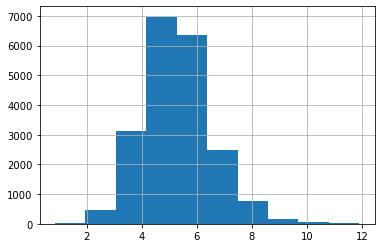We can have a similar reasoning for the number of people living in a house. Let's check the number of districts, where the AveOccup is greater than 13:

data_df[data_df['AveOccup']>13]

Output:
MedInc HouseAge AveRooms AveBedrms Population AveOccup Latitude Longitude AvePropVal
1039 4.3029 16.0 6.090592 1.121951 4930.0 17.177700 38.42 -120.97 1.21900
3364 5.5179 36.0 5.142857 1.142857 4198.0 599.714286 40.41 -120.51 0.67500
4479 3.5769 41.0 4.940000 1.100000 925.0 18.500000 34.07 -118.16 1.35700
5986 1.8750 52.0 4.500000 1.206349 2688.0 21.333333 34.10 -117.71 2.12500
7164 2.1845 36.0 4.812155 1.110497 2862.0 15.812155 34.06 -118.17 1.23800
8874 9.3370 52.0 7.285714 1.214286 1154.0 41.214286 34.06 -118.45 5.00001
9172 4.2391 5.0 5.123810 0.933333 8733.0 83.171429 34.47 -118.59 1.54600
12104 1.6250 8.0 7.600000 0.950000 1275.0 63.750000 33.97 -117.33 1.62500
13034 6.1359 52.0 8.275862 1.517241 6675.0 230.172414 38.69 -121.15 2.25000
13366 4.2578 36.0 5.258824 1.117647 2886.0 33.952941 33.94 -117.63 1.83300
14756 2.5625 23.0 4.589091 1.069091 5176.0 18.821818 32.56 -116.97 1.53300
15790 4.3958 52.0 2.117647 0.926471 1061.0 15.602941 37.77 -122.40 2.25000
16420 5.7485 26.0 5.366667 0.900000 1542.0 51.400000 37.89 -121.29 1.62500
16643 1.0298 20.0 2.398467 0.812261 3574.0 13.693487 35.32 -120.65 3.00000
16669 4.2639 46.0 9.076923 1.307692 6532.0 502.461538 35.32 -120.70 3.50000
16672 1.6563 16.0 5.125000 0.937500 542.0 16.937500 35.34 -120.69 0.42500
18520 2.4732 14.0 4.445402 1.074713 4731.0 13.594828 37.00 -122.06 1.31300
19006 10.2264 45.0 3.166667 0.833333 7460.0 1243.333333 38.32 -121.98 1.37500
19435 0.5360 16.0 2.111111 2.111111 166.0 18.444444 37.67 -121.04 1.62500
19524 0.9573 19.0 2.951220 1.000000 658.0 16.048780 37.64 -121.00 1.62500
20121 3.1719 8.0 6.979221 1.285714 5087.0 13.212987 37.79 -120.45 1.15400
20352 4.0208 8.0 2.500000 0.625000 309.0 19.312500 34.22 -119.09 0.52500
data_df['AveOccup'][data_df['AveOccup']<=13].hist()

Output:
<AxesSubplot:>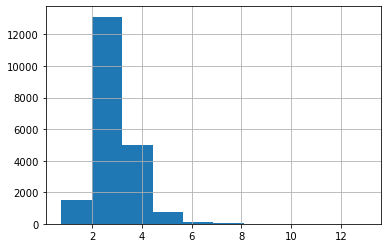Let's have a look at all the feature histograms:

data_df.hist(bins=50, figsize=(15,15))
plt.show()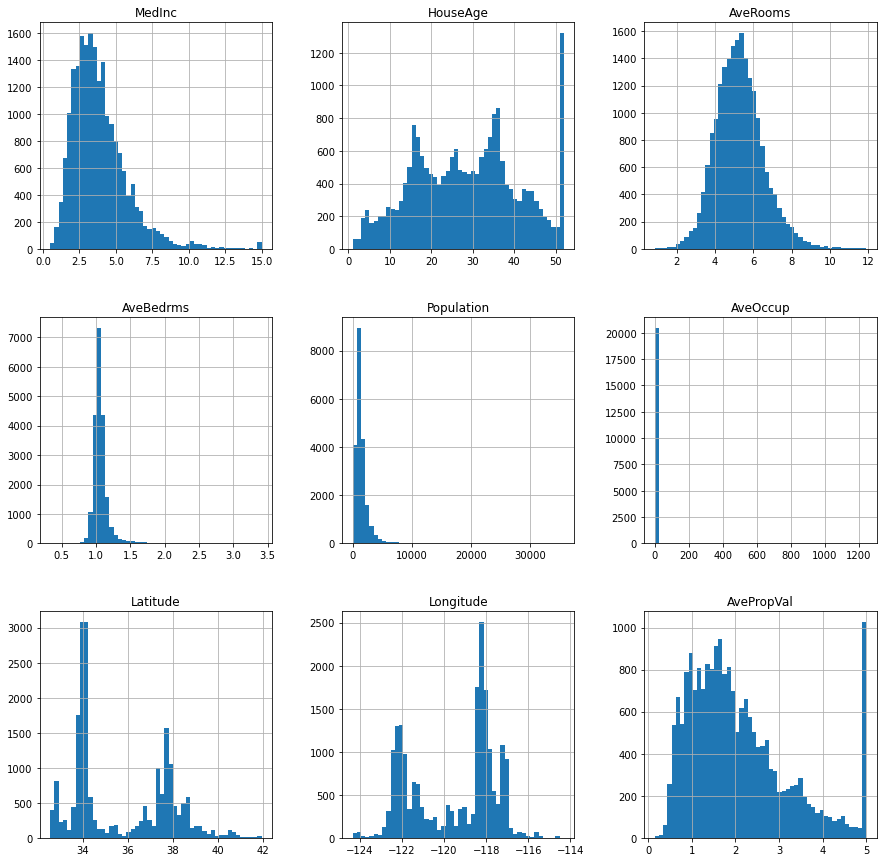from pandas.plotting import scatter_matrix
attributes = ['HouseAge', 'MedInc',
'AveRooms', 'AveOccup']
scatter_matrix(data_df[attributes], figsize=(12,8));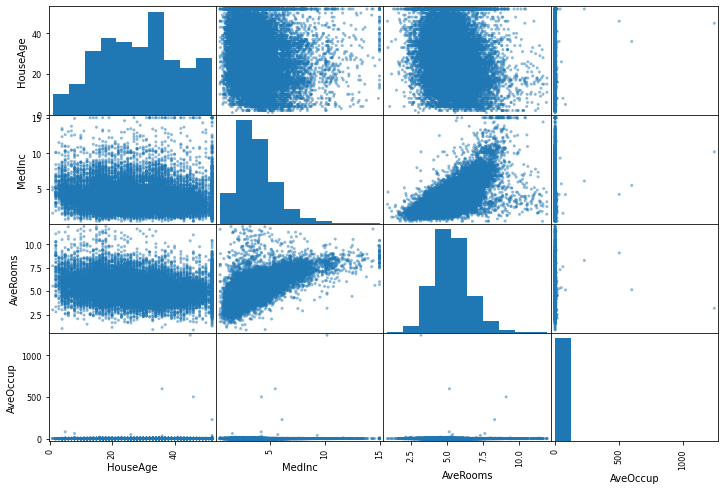data_df

Output:
MedInc HouseAge AveRooms AveBedrms Population AveOccup Latitude Longitude AvePropVal
0 8.3252 41.0 6.984127 1.023810 322.0 2.555556 37.88 -122.23 4.526
1 8.3014 21.0 6.238137 0.971880 2401.0 2.109842 37.86 -122.22 3.585
2 7.2574 52.0 8.288136 1.073446 496.0 2.802260 37.85 -122.24 3.521
3 5.6431 52.0 5.817352 1.073059 558.0 2.547945 37.85 -122.25 3.413
4 3.8462 52.0 6.281853 1.081081 565.0 2.181467 37.85 -122.25 3.422
... ... ... ... ... ... ... ... ... ...
20635 1.5603 25.0 5.045455 1.133333 845.0 2.560606 39.48 -121.09 0.781
20636 2.5568 18.0 6.114035 1.315789 356.0 3.122807 39.49 -121.21 0.771
20637 1.7000 17.0 5.205543 1.120092 1007.0 2.325635 39.43 -121.22 0.923
20638 1.8672 18.0 5.329513 1.171920 741.0 2.123209 39.43 -121.32 0.847
20639 2.3886 16.0 5.254717 1.162264 1387.0 2.616981 39.37 -121.24 0.894

20495 rows × 9 columns

data_df.plot(kind='scatter', x='Population', y='AvePropVal',
alpha=0.1, figsize=(8,5))

Output:
<AxesSubplot:xlabel='Population', ylabel='AvePropVal'>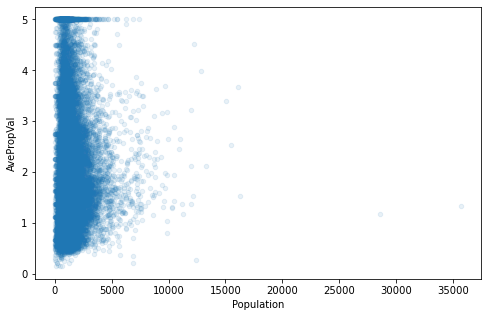### Splitting the Data

from sklearn.model_selection import train_test_split
data_sets = train_test_split(dataset.data,
dataset.target,
test_size=0.30,
random_state=42)

data_train, data_test, targets_train, targets_test = data_sets


### A First Classifier

clf = MLPRegressor(solver='lbfgs',        #  ‘lbfgs’, ‘sgd’, ‘adam’ (default)
alpha=1e-5,            # used for regularization, ovoiding overfitting by penalizing large magnitudes
hidden_layer_sizes=(10, 2),
activation='logistic', # ‘identity’, ‘logistic’, ‘tanh’, ‘relu’ (default)
max_iter=10000,
random_state=42)
clf.fit(data_train, targets_train)
clf.predict(data_train)

Output:
array([2.03631477, 2.02460928, 2.15315216, ..., 1.70455007, 2.31221342,
2.57457578])

Parameters:

• activation{‘identity’, ‘logistic’, ‘tanh’, ‘relu’}, default=’relu’ Activation function for the hidden layer.

• ‘identity’, no-op activation, useful to implement linear bottleneck, returns f(x) = x
• ‘logistic’, the logistic sigmoid function, returns f(x) = 1 / (1 + exp(-x)).
• ‘tanh’, the hyperbolic tan function, returns f(x) = tanh(x).
• ‘relu’, the rectified linear unit function, returns f(x) = max(0, x)

• ‘lbfgs’ is an optimizer in the family of quasi-Newton methods.
• ‘sgd’ refers to stochastic gradient descent.
• ‘adam’ refers to a stochastic gradient-based optimizer proposed by Kingma, Diederik, and Jimmy Ba

Note: The default solver ‘adam’ works pretty well on relatively large datasets (with thousands of training samples or more) in terms of both training time and validation score. For small datasets, however, ‘lbfgs’ can converge faster and perform better.

### Data Preprocessing

#### Removing Outliers

max_ave_rooms = 12
# column with index 2 corresponds to average number of rooms
shape = dataset.data.shape
cleansed_shape = dataset.data[dataset.data[:,2] <= max_ave_rooms].shape
print(shape, cleansed_shape)
n_outliers = shape-cleansed_shape
print(f"Number of outliers, more than {max_ave_rooms} bedrooms: {n_outliers}")

(20640, 8) (20495, 8)
Number of outliers, more than 12 bedrooms: 145


Let us remove all data with an average number of rooms greater than max_ave_rooms:

x = dataset.data[:,2] <= max_ave_rooms  # Boolean array
data = dataset.data[x]
targets = dataset.target[x]
data.shape, targets.shape

Output:
((20495, 8), (20495,))
data.shape

Output:
(20495, 8)

Before we go on like this, let us have a look at the statistics for each feature:

data_df.describe()

Output:
MedInc HouseAge AveRooms AveBedrms Population AveOccup Latitude Longitude AvePropVal
count 20495.000000 20495.000000 20495.000000 20495.000000 20495.000000 20495.000000 20495.000000 20495.000000 20495.000000
mean 3.870574 28.715540 5.297038 1.069902 1432.511979 3.074511 35.621918 -119.573349 2.071016
std 1.898052 12.575564 1.274305 0.143758 1132.726950 10.422249 2.130666 2.003646 1.154817
min 0.499900 1.000000 0.846154 0.333333 3.000000 0.750000 32.540000 -124.350000 0.149990
25% 2.562500 18.000000 4.434997 1.005747 793.000000 2.432727 33.930000 -121.800000 1.198000
50% 3.534700 29.000000 5.219585 1.048128 1171.000000 2.823322 34.250000 -118.490000 1.801000
75% 4.744800 37.000000 6.027555 1.098000 1729.000000 3.285983 37.710000 -118.010000 2.652000
max 15.000100 52.000000 11.901869 3.411111 35682.000000 1243.333333 41.950000 -114.310000 5.000010
# "MedInc", "HouseAge", "AveRooms", "AveBedrms", "Population", "AveOccup", "Latitude", "Longitude"

x = data[:,5] <= 10   # AveOccup
data = data[x]
targets = targets[x]
data.shape, targets.shape

Output:
((20459, 8), (20459,))
avebedrms_index = 3

np.min(data[:,3]), np.max(data[:,3])

Output:
(0.3333333333333333, 3.411111111111111)
np.max(dataset.data[:3])

Output:
2401.0

The outliers for this feature have already disappeared due to the previous cleaning actions.

#### Another try on classification:

The original data:

from sklearn.model_selection import train_test_split
data_sets = train_test_split(data,
targets,
test_size=0.30,
random_state=42)

data_train, data_test, targets_train, targets_test = data_sets


The cleansed data:

from sklearn.model_selection import train_test_split
data_sets = train_test_split(dataset.data,
dataset.target,
test_size=0.30,
random_state=42)

data_train2, data_test2, targets_train2, targets_test2 = data_sets

data.shape

Output:
(20459, 8)
data_train.shape, data_train2.shape

Output:
((14321, 8), (14448, 8))
clf = MLPRegressor(solver='lbfgs',        #  ‘lbfgs’, ‘sgd’, ‘adam’ (default)
alpha=1e-5,            # used for regularization, ovoiding overfitting by penalizing large magnitudes
hidden_layer_sizes=(10, 2),
activation='logistic', # ‘identity’, ‘logistic’, ‘tanh’, ‘relu’ (default)
max_iter=10000,
random_state=42)
clf.fit(data_train, targets_train)
print(clf.score(data_train, targets_train))
print(clf.score(data_test, targets_test))

0.6529314309501919
0.6429262961386791


We can see that classifying with uncleansed data gives us slightly worse results:

clf = MLPRegressor(solver='lbfgs',        #  ‘lbfgs’, ‘sgd’, ‘adam’ (default)
alpha=1e-5,            # used for regularization, ovoiding overfitting by penalizing large magnitudes
hidden_layer_sizes=(10, 2),
activation='logistic', # ‘identity’, ‘logistic’, ‘tanh’, ‘relu’ (default)
max_iter=10000,
random_state=42)
clf.fit(data_train2, targets_train2)
print(clf.score(data_train2, targets_train2))
print(clf.score(data_test2, targets_test2))

0.6497290839847478
0.643381317467951


#### Scaling the Data

As the data varies a lot in magnitude, we can or should scale the data:

from sklearn import preprocessing

data_scaled = preprocessing.scale(data)
data_scaled.shape

Output:
(20459, 8)
data_scaled[:5]

Output:
array([[ 2.35060892,  0.97741648,  1.32614432, -0.32120816, -0.98212191,
-0.47819706,  1.06028422, -1.32627682],
[ 2.33805042, -0.61364072,  0.73967535, -0.68360646,  0.86018141,
-1.06008696,  1.05089666, -1.32128549],
[ 1.78716513,  1.85249794,  2.35130642,  0.02519331, -0.82793203,
-0.15611866,  1.04620287, -1.33126814],
[ 0.93535084,  1.85249794,  0.40886977,  0.02249278, -0.7729908 ,
-0.48813255,  1.04620287, -1.33625946],
[-0.01281562,  1.85249794,  0.77404337,  0.07847414, -0.76678776,
-0.96657835,  1.04620287, -1.33625946]])
data[:5]

Output:
array([[ 8.32520000e+00,  4.10000000e+01,  6.98412698e+00,
1.02380952e+00,  3.22000000e+02,  2.55555556e+00,
3.78800000e+01, -1.22230000e+02],
[ 8.30140000e+00,  2.10000000e+01,  6.23813708e+00,
9.71880492e-01,  2.40100000e+03,  2.10984183e+00,
3.78600000e+01, -1.22220000e+02],
[ 7.25740000e+00,  5.20000000e+01,  8.28813559e+00,
1.07344633e+00,  4.96000000e+02,  2.80225989e+00,
3.78500000e+01, -1.22240000e+02],
[ 5.64310000e+00,  5.20000000e+01,  5.81735160e+00,
1.07305936e+00,  5.58000000e+02,  2.54794521e+00,
3.78500000e+01, -1.22250000e+02],
[ 3.84620000e+00,  5.20000000e+01,  6.28185328e+00,
1.08108108e+00,  5.65000000e+02,  2.18146718e+00,
3.78500000e+01, -1.22250000e+02]])

#### Generating Polynomial Features

from sklearn.preprocessing import PolynomialFeatures


Polynomial Features:

PolynomialFeatures does the following: Generate a new feature matrix consisting of all polynomial combinations of the features with degree less than or equal to the specified degree. For example, if an input sample is two dimensional and of the form $[a, b]$, the degree-2 polynomial features are $[1, a, b, a^2, ab, b^2]$.

import numpy as np
from sklearn.preprocessing import PolynomialFeatures
X = np.arange(6).reshape(3, 2)
X

Output:
array([[0, 1],
[2, 3],
[4, 5]])
poly = PolynomialFeatures(degree=2)
poly.fit_transform(X)

Output:
array([[ 1.,  0.,  1.,  0.,  0.,  1.],
[ 1.,  2.,  3.,  4.,  6.,  9.],
[ 1.,  4.,  5., 16., 20., 25.]])

If the interaction_only parameter is set to True, only interaction features are produced: features that are products of at most degree distinct input features (so not x 2, x * x 3, etc.).

poly = PolynomialFeatures(interaction_only=True)
poly.fit_transform(X)

Output:
array([[ 1.,  0.,  1.,  0.],
[ 1.,  2.,  3.,  6.],
[ 1.,  4.,  5., 20.]])

Let's get back to our housing data:

pft = PolynomialFeatures(degree=2)
data_poly = pft.fit_transform(data_scaled)
data_poly
data_poly

Output:
array([[ 1.        ,  2.35060892,  0.97741648, ...,  1.12420263,
-1.40623038,  1.7590102 ],
[ 1.        ,  2.33805042, -0.61364072, ...,  1.10438378,
-1.38853451,  1.74579536],
[ 1.        ,  1.78716513,  1.85249794, ...,  1.09454046,
-1.39277656,  1.77227486],
...,
[ 1.        , -1.14529647, -0.93185216, ...,  3.19630178,
-1.46986232,  0.67593593],
[ 1.        , -1.0570704 , -0.8522993 , ...,  3.19630178,
-1.5590982 ,  0.7604999 ],
[ 1.        , -0.78194435, -1.01140502, ...,  3.09639525,
-1.46427431,  0.69245011]])

### Splitting our Dataset

from sklearn.model_selection import train_test_split
data_sets = train_test_split(data_poly,
targets,
test_size=0.30,
random_state=42)

data_train, data_test, targets_train, targets_test = data_sets


### Creating and Training a Classifier

clf = MLPRegressor(solver='lbfgs',   #  ‘lbfgs’, ‘sgd’, ‘adam’ (default)
alpha=1e-5,     # used for regularization, ovoiding overfitting by penalizing large magnitudes
hidden_layer_sizes=(5, 2),
activation='relu', # ‘identity’, ‘logistic’, ‘tanh’, ‘relu’ (default)
max_iter=10000,
early_stopping=True,
random_state=42)
clf.fit(data_train, targets_train)
print(clf.score(data_train, targets_train))
print(clf.score(data_test, targets_test))

0.7952528759049259
0.7752743785730409

clf.predict(data_train)

Output:
array([2.56172046, 2.35781579, 1.92601089, ..., 2.307256  , 3.44872006,
2.85340133])
targets_train

Output:
array([2.109, 2.257, 1.508, ..., 3.1  , 2.825, 2.42 ])`